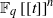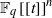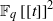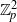Home

# KAKEYA SETS OVER NON-ARCHIMEDEAN LOCAL RINGS

## Abstract

In a recent paper of Ellenberg, Oberlin, and Tao [The Kakeya set and maximal conjectures for algebraic varieties over finite fields. Mathematika 56 (2010), 1–25], the authors asked whether there are Besicovitch phenomena in${ \mathbb{F} }_{q} \mathop{[[t] ] }\nolimits ^{n}$ . In this paper, we answer their question in the affirmative by explicitly constructing a Kakeya set of measure zero in${ \mathbb{F} }_{q} \mathop{[[t] ] }\nolimits ^{n}$ . Furthermore, we prove that any Kakeya set in${ \mathbb{F} }_{q} \mathop{[[t] ] }\nolimits ^{2}$ or${ \mathbb{Z} }_{p}^{2}$ is of Minkowski dimension 2.

## References

Hide All
MathJax
MathJax is a JavaScript display engine for mathematics. For more information see http://www.mathjax.org.

# KAKEYA SETS OVER NON-ARCHIMEDEAN LOCAL RINGS

## Metrics

### Full text viewsFull text views reflects the number of PDF downloads, PDFs sent to Google Drive, Dropbox and Kindle and HTML full text views.

Total number of HTML views: 0
Total number of PDF views: 0 *Loading metrics...

### Abstract viewsAbstract views reflect the number of visits to the article landing page.

Total abstract views: 0 *Loading metrics...

* Views captured on Cambridge Core between <date>. This data will be updated every 24 hours.

Usage data cannot currently be displayed